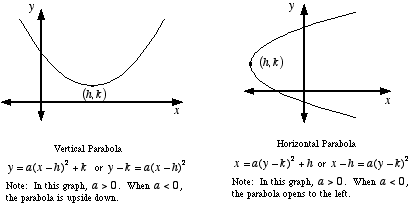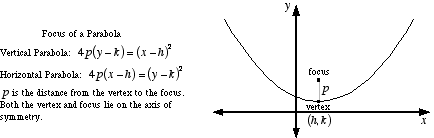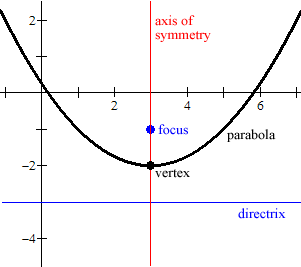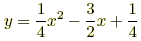# parabola

Parabola

A u-shaped curve with certain specific properties. Formally, a parabola is defined as follows: For a given point, called the focus, and a given line not through the focus, called the directrix, a parabola is the locus of points such that the distance to the focus equals the distance to the directrix.

Note: It is a common error to call any u-shaped curve a parabola. A parabola must satisfy the conditions listed above, and a parabola always has a quadratic equation.Example:This is a graph of the parabolawith all its major features labeled: axis of symmetry, focus, vertex, and directrix.